## Airy Differential Equation

Some authors define a general Airy differential equation as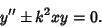(1)

This equation can be solved by series solution using the expansions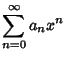(2)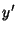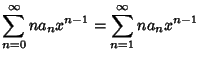(3)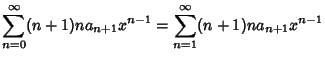(4)

Specializing to the conventional'' Airy differential equation occurs by taking the Minus Sign and setting. Then plug (4) into(5)

to obtain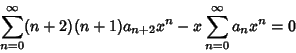(6)(7)(8)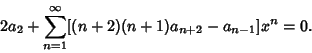(9)

In order for this equality to hold for all, each term must separately be 0. Therefore,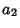(10)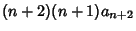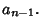(11)

Starting with theterm and using the above Recurrence Relation, we obtain(12)

Continuing, it follows by Induction that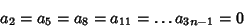(13)

for, 2, .... Now examine terms of the form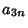.(14)(15)(16)

Again by Induction,(17)

for, 2, .... Finally, look at terms of the form,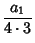(18)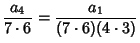(19)(20)

By Induction,(21)

for, 2, .... The general solution is therefore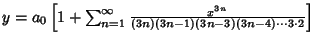(22)
For a general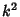with a Minus Sign, equation (1) is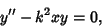(23)

and the solution is(24)

whereis a Modified Bessel Function of the First Kind. This is usually expressed in terms of the Airy Functions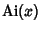and(25)

If the Plus Sign is present instead, then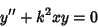(26)

and the solutions are(27)

where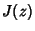is a Bessel Function of the First Kind.

See also Airy-Fock Functions, Airy Functions, Bessel Function of the First Kind, Modified Bessel Function of the First Kind